# Transcendental extension

(diff) ← Older revision | Latest revision (diff) | Newer revision → (diff)

A field extension that is not algebraic (cf. Extension of a field). An extension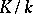is transcendental if and only if the fieldcontains elements that are transcendental over, that is, elements that are not roots of any non-zero polynomial with coefficients in.

The elements of a set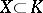are called algebraically independent overif for any finite set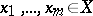and any non-zero polynomial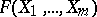with coefficients in,The elements ofare transcendental over. Ifis a maximal set of algebraically independent elements over, thenis called a transcendence basis ofover. The cardinality ofis called the transcendence degree ofoverand is an invariant of the extension. For a tower of fields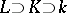, the transcendence degree of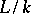is equal to the sum of the transcendence degrees of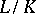and.

If all elements of a setare algebraically independent over, then the extensionis called purely transcendental. In this case the fieldis isomorphic to the field of rational functions in the set of variablesover. Any field extensioncan be represented as a tower of extensions, whereis an algebraic andis a purely transcendental extension. Ifcan be chosen so thatis a separable extension, then the extensionis called separably generated, and the transcendence basis ofoveris called a separating basis. Ifis separably generated over, thenis separable over. In the case when the extensionis finitely generated, the converse holds as well. By definition, an extensionis separable if and only if any derivation (cf. Derivation in a ring) ofextends to. Such an extension is uniquely determined for any derivation if and only if the extensionis algebraic.

How to Cite This Entry:
Transcendental extension. Encyclopedia of Mathematics. URL: http://encyclopediaofmath.org/index.php?title=Transcendental_extension&oldid=17184
This article was adapted from an original article by L.V. Kuz'min (originator), which appeared in Encyclopedia of Mathematics - ISBN 1402006098. See original article